# Introduction

At this stage in your `R` training, you’ve covered quite a lot of ground. You know the basics of:

• Exploring your data with summary statistics and visualizations;
• Engineering new features that capture meaningful signal in your data
• Modeling your data and evaluating the models.

In today’s session, we’ll synthesize and build on some of these skills by identifying a cause of an odd spike in AirBnB rental prices. We’ll practice our data manipulation and visualization skills, perform some very basic time-series analysis, and learn to think about data analysis as a process of stripping away different layers of signal in your data. Throughout our discussion, the focus will be on using the “tidy” tools like `dplyr`, `tidyr`, and `ggplot2` you learned in Session 2 in the context of an advanced data investigation task that incorporates modeling, complex feature engineering, and visualization.

In terms of software tools in `R`, we’ll:

1. Review elementary data reading and preparation.
2. Review some basic plotting with `ggplot2`.
3. Fit many models at once using `tidyr`, `dplyr` and a bit of help from functional programming tools.
4. Use grouped `mutate` to efficiently construct complex columns.
5. Do some elementary geographic visualization with `ggmap`.

# Before the Session

You need to ensure that the `tidyverse` packages is installed. You will also need the `ggmap` package:

``````install.packages('tidyverse')
install.packages('ggmap')``````

You should already have installed these tools in Sessions 2 and 3, so if you have attended those sessions and successfully run the code, you don’t need to do anything else.

Let’s get started!

# Setup

``````library(tidyverse)
library(broom)
library(ggmap)
library(lubridate)
library(stringr)``````

For today’s exploration, we’ll need the `calendar` and `listing` data sets.

``````calendar <- read_csv('data/calendar.csv')

## Data Preparation

We’re going to be studying temporal trends in AirBnB prices today, so our first step will be to generate the appropriate set of features. To account for the fact that some rentals are larger than others, our main feature will be the listed price per person over time. To capture the temporal aspect, we’ll base our data set on the `calendar` data, but we’ll need to grab the `accommodates` column from `listings`:

``````prices <- calendar %>%
left_join(listings, by = c('listing_id' = 'id' )) %>%
select(listing_id, date, price = price.x, accommodates)

``````## # A tibble: 5 × 4
##   listing_id       date price accommodates
##        <int>     <date> <chr>        <int>
## 1   12147973 2017-09-05  <NA>            4
## 2   12147973 2017-09-04  <NA>            4
## 3   12147973 2017-09-03  <NA>            4
## 4   12147973 2017-09-02  <NA>            4
## 5   12147973 2017-09-01  <NA>            4``````

Looks good. Next, let’s construct the `price_per` column. First, we’ll need to convert the `price` column into a number:

``````prices <- prices %>%
mutate(price = as.numeric(sub("\\\$|","", price)),
price_per = price / accommodates) %>%
select(listing_id, date, price_per) ``````
``````## Warning in eval(substitute(expr), envir, enclos): NAs introduced by
## coercion``````
``prices %>% head(5)``
``````## # A tibble: 5 × 3
##   listing_id       date price_per
##        <int>     <date>     <dbl>
## 1   12147973 2017-09-05        NA
## 2   12147973 2017-09-04        NA
## 3   12147973 2017-09-03        NA
## 4   12147973 2017-09-02        NA
## 5   12147973 2017-09-01        NA``````

## Last bit of data preparation

Now let’s do a bit of cleaning. In order, we’re going to:

• Remove rows with `NA` in any entries.
• Remove rows corresponding to listings that have less than 200 days of data
• Change the date to a format that will be more workable for later modeling.
``````prices <- prices %>%
mutate(date = as.numeric(date),
listing_id = as.character(listing_id)) %>%
na.omit() %>%
group_by(listing_id) %>%
filter(n() >= 200) ``````

# Preliminary Explorations

Let’s take a quick look at what we have. First, we’ll visualize a few sample time series.1 The `head` function takes only the first 2000 rows of data, which prevents overplotting.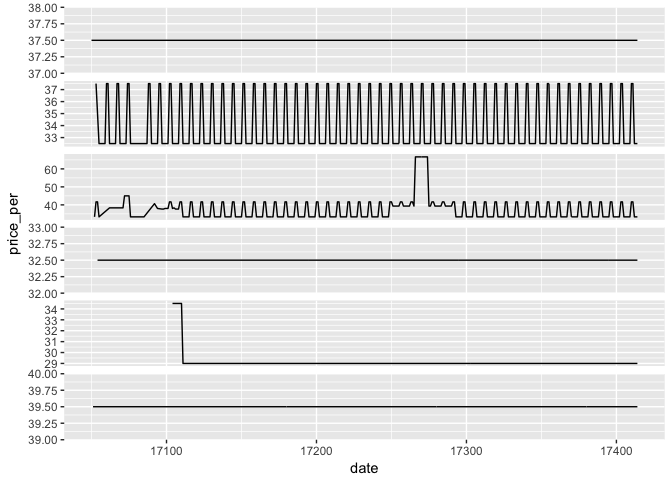Sample time series.

``````prices %>%
ggplot() +
aes(x = date,
y = price_per,
group = listing_id) +
geom_line() +
facet_wrap(~listing_id,
ncol = 1,
scales = 'free_y') +
theme(strip.text.x = element_blank())``````

Looks like there’s a lot of variety: some prices are simply constant; others vary periodically; and others still have specific upticks at certain times of day. We can get “the big picture” by plotting the average over time: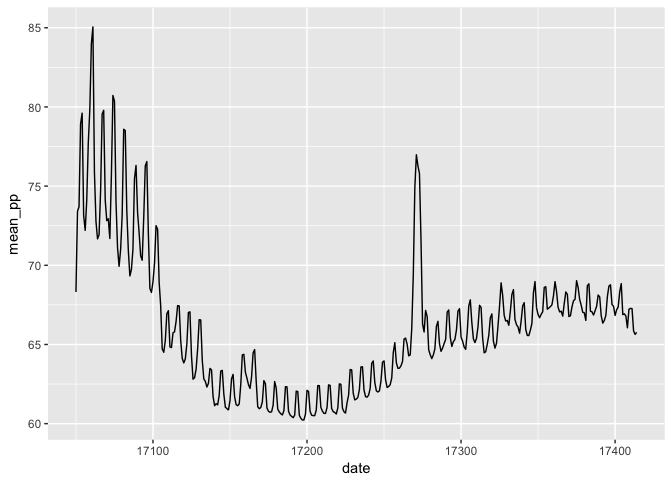Average over time.

``````mean_plot <- prices %>%
group_by(date) %>%
summarise(mean_pp = mean(price_per, na.rm = T)) %>%
ggplot() +
aes(x = date, y = mean_pp) +
geom_line()
mean_plot ``````

While inspecting means isn’t usually enough to give you the full picture, it can help get you oriented. Just from the mean series , we can observe at least three phenomena:

1. Long-term (seasonal?) variation, reflected by the “overal shape” of the chart.
2. Periodic oscillation – on average, weekends are more expensive than weekdays.
3. A prominent spike around time 17300, which happens to fall in April.

We can think of each of these phenomena as a “signal” – something interesting that the data may be trying to tell us. The remainder of our time together will focus on isolating these different components, to study them separately. We’ll do this by peeling back layers: first identifying and subtracting the long-term trend; then identifying and subtracting the periodic oscillation; and finally studying the spike itself in relative isolation. Of course, data is messy and we won’t be able to carry out this program perfectly. As we’ll see, though, once we’re done, we’ll be able to “see” the reason for the spike in an intuitive way.

# Capturing Long-Term Trend

We’d like to begin modeling our data by fitting a function that captures the long-term trend. There’s considerable diversity in the different listings, and we shouldn’t expect a single model to accurately model each series. We’d therefore like to construct a model for each series.

So, we have two questions:

1. What model should we use?
2. How should we fit a model per series?

Model choice: there are lots of good answers in the theory of time-series analysis.2 The simplest non-parameteric model is to take a moving average, but auto-regressive models give parameteric representations Since you now have some background in machine learning, we’ll use a simple non-parameteric regression smoother, called “LOcal regrESSion,” usually abbreviated to “LOESS.” We can easily visualize a LOESS model, since it’s `ggplot2`’s default for `geom_smooth()`: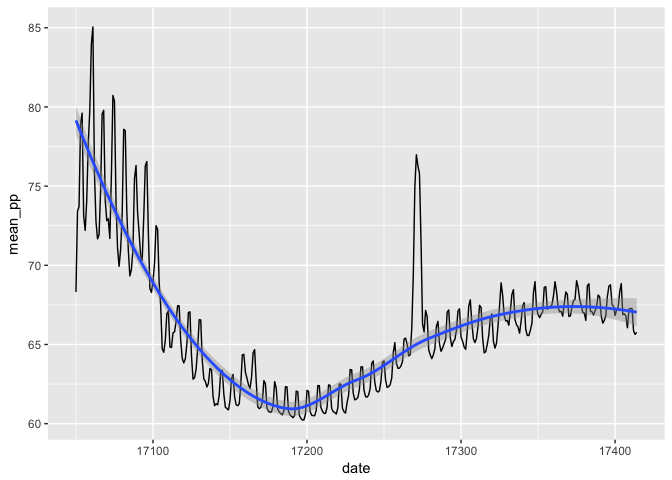Average over time with a LOESS smoother.

``````mean_plot +
geom_smooth() ``````
``## `geom_smooth()` using method = 'loess'``

Apparently, the LOESS model captures the overall seasonality quite nicely. It won’t look quite this clean on individual level data, as we’ll see in a moment.

Fitting many models: We have 1698 distinct series to model. How should we proceed? One approach is a for-loop, but `R` hates those. There are plenty of alternatives,3 Such as using the `by()` function, or manually writing a complex function that can be used in in a `mutate` call. but we’ll use a particularly elegant method that uses tools you already learned in Session 2. It starts by doing something quite strange.

## Data Frames of Data Frames…

Our key tool is the function `tidyr::nest()`:4 the argument `-listing_id` tells `nest` not to fold in the `listing_id`, but to construct a separate data frame for each one. `nest(-listing_id)` is a lot like `group_by(listing_id)`, but instead of a group of rows for each id, you have a single row containing a data frame.

``````prices_nested <- prices %>%
nest(-listing_id)``````

The function `nest` converts our perfectly well-behaved data frame into something funky: a data frame of data frames:5 The output below states that the contents of the second column have class `tibble`. A `tibble` is a slightly gussied up version of a `data.frame`, and I’ll just refer to them as “data frames” from here on out.

``prices_nested %>% head(5)``
``````## # A tibble: 5 × 2
##   listing_id               data
##        <chr>             <list>
## 1    3075044 <tibble [359 × 2]>
## 2       6976 <tibble [319 × 2]>
## 3    7651065 <tibble [334 × 2]>
## 4    5706985 <tibble [344 × 2]>
## 5    2843445 <tibble [365 × 2]>``````

By inspecting an element of the `data` column, we can confirm it’s a data frame:

``prices_nested\$data[] %>% head(5)``
``````## # A tibble: 5 × 2
##    date price_per
##   <dbl>     <dbl>
## 1 17400      32.5
## 2 17399      32.5
## 3 17398      32.5
## 4 17397      37.5
## 5 17396      37.5``````

Ok, why on earth would we want this? Any ideas?

The key point is that we can use knowledge you already have to fit our models. Specifically,

We can now `mutate` with functions whose arguments are data frames.

This probably isn’t clear yet, but we’ll see this become quite powerful.

Recall that a `mutate` call looks like this:

``````data %>%
mutate(new_col = some_function(existing_col))``````

Since we have a column containing data, we can use `mutate` to construct a new column of models – all we need to do is write a simple function that constructs a LOESS model from data. We haven’t discussed writing functions yet, but the idea is simple:

``````my_loess <- function(data, span){
loess(price_per ~ date,
data = data,
na.action = na.exclude,
span = span)
}``````

In words,

`my_loess` now refers to a function whose arguments are `data` and `span`. The action of `my_loess` is to return a `loess` object, using `data` and `span` as arguments.

With that done, we are ready to fit 1698 models with one simple call to `mutate`. We can even specify the “span” of the model manually. However, the most direct approach won’t work:

``````prices_with_models <- prices_nested %>%
mutate(model = my_loess(data, span = .5))``````
``## Error in eval(expr, envir, enclos): arguments imply differing number of rows: 359, 319, 334, 344, 365, 347, 343, 341, 339, 349, 295, 262, 332, 207, 299, 356, 310, 353, 325, 362, 300, 351, 361, 355, 254, 306, 267, 260, 248, 258, 348, 333, 259, 331, 328, 301, 340, 250, 346, 337, 363, 255, 316, 358, 309, 279, 285, 257, 276, 290, 364, 292, 240, 327, 283, 335, 266, 284, 338, 213, 211, 314, 305, 298, 308, 302, 288, 286, 209, 296, 224, 326, 311, 201, 304, 252, 320, 350, 303, 294, 217, 322, 360, 231, 317, 274, 323, 352, 330, 263, 282, 242, 307, 329, 280, 289, 281, 297, 208, 245, 324, 237, 206, 312, 318, 336, 313, 321, 357, 293, 225, 315, 291, 241, 244, 251, 249, 253, 273, 246, 270, 227, 354, 342, 345, 261, 272, 223, 278, 264, 275, 203, 235, 271, 236, 204, 216, 233, 277, 269, 222, 215, 219, 247, 238, 200, 268, 243, 226, 228, 230, 287, 256, 234, 210, 214, 218``

The wrinkle is that we need to use `purrr::map` to vectorize the function `my_loess`.6 The `purrr` package is a useful package, also by Hadley Wickham, for easy functional programming in `R`. Vectorization is one of the key concepts of functional programming. A vectorized function acts on an entire column of a data frame at once; `dplyr::mutate` requires that all functions it uses be vectorized. Almost all the basic functions you know, like `sum()`, `mean()`, `paste`, etc. are vectorized, so this problem may not have come up yet.

Other than the manual vectorization with `purrr::map`, our model fitting now looks like any other `mutate` call:

``````prices_with_models <- prices_nested %>%
mutate(model = map(data, my_loess, span = .25))``````

Ok, so what does the `model` column of `prices_with_models` now contain?

``prices_with_models %>% head(5)``
``````## # A tibble: 5 × 3
##   listing_id               data       model
##        <chr>             <list>      <list>
## 1    3075044 <tibble [359 × 2]> <S3: loess>
## 2       6976 <tibble [319 × 2]> <S3: loess>
## 3    7651065 <tibble [334 × 2]> <S3: loess>
## 4    5706985 <tibble [344 × 2]> <S3: loess>
## 5    2843445 <tibble [365 × 2]> <S3: loess>``````

Just like there are data frames in the `data` column, there are now statistical models in the `model` column. Let’s take a closer look:

``summary(prices_with_models\$model[])``
``````## Call:
## loess(formula = price_per ~ date, data = data, na.action = na.exclude,
##     span = span)
##
## Number of Observations: 359
## Equivalent Number of Parameters: 12.05
## Residual Standard Error: 2.294
## Trace of smoother matrix: 13.31  (exact)
##
## Control settings:
##   span     :  0.25
##   degree   :  2
##   family   :  gaussian
##   surface  :  interpolate      cell = 0.2
##   normalize:  TRUE
##  parametric:  FALSE
## drop.square:  FALSE``````

Yup, looks like a model! But just having the model itself isn’t so interesting – we’d like to compare the data to the model predictions and inspect the model residuals. We can add a new column that contains all this information using the `broom::augment` function.7 The `broom` package “tidies” model outputs by converting them into data frames. This allows us to do complex modeling tasks without leaving our standard `dplyr` pipelines. Just like before, we need to vectorize with `purrr::map`:

``````prices_with_preds <- prices_with_models %>%
mutate(preds = map(model, augment))``````

What does the result look like?

``prices_with_preds\$preds[] %>% tbl_df() %>% head(5)``
``````## # A tibble: 5 × 5
##   price_per  date  .fitted   .se.fit    .resid
##       <dbl> <dbl>    <dbl>     <dbl>     <dbl>
## 1      32.5 17400 33.89916 0.3889374 -1.399161
## 2      32.5 17399 33.90330 0.3785467 -1.403301
## 3      32.5 17398 33.90722 0.3703511 -1.407225
## 4      37.5 17397 33.91094 0.3642069  3.589062
## 5      37.5 17396 33.91444 0.3599486  3.585556``````

Each entry in the column `preds` contains the original data (`price_per` and `date`), as well as the `.fitted` predictions, the standard error `.se.fit` associated with the model prediction, and the model residual `.resid`, which is simply the difference between `price_per` and `.fitted`. As always in statistics, the residuals are extremely important. We can think of them as data minus current signal: they are what the data still has to tell us after we’ve extracted the seasonal trend signals.

## Back to Sanity

Ok, let’s get out of this crazy data frames of data frames business.8 `unnest` is the inverse of `nest`; its argument says which column of data frames you wish to access.

We’ll also convert the date back into a more human-readable format:

``````prices_modeled <- prices_with_preds %>%
unnest(preds) %>%
mutate(date = as.Date.numeric(date,
origin = "1970-01-01"))
``````## # A tibble: 5 × 6
##   listing_id price_per       date  .fitted   .se.fit    .resid
##        <chr>     <dbl>     <date>    <dbl>     <dbl>     <dbl>
## 1    3075044      32.5 2017-08-22 33.89916 0.3889374 -1.399161
## 2    3075044      32.5 2017-08-21 33.90330 0.3785467 -1.403301
## 3    3075044      32.5 2017-08-20 33.90722 0.3703511 -1.407225
## 4    3075044      37.5 2017-08-19 33.91094 0.3642069  3.589062
## 5    3075044      37.5 2017-08-18 33.91444 0.3599486  3.585556``````

Notice what’s happened: we now have a single data frame with no nested structure. We also have our `listing_id` column back, as well as the original data and the model predictions. This means that we’re ready to start visualizing the fit and evaluating its quality. Let’s take a look!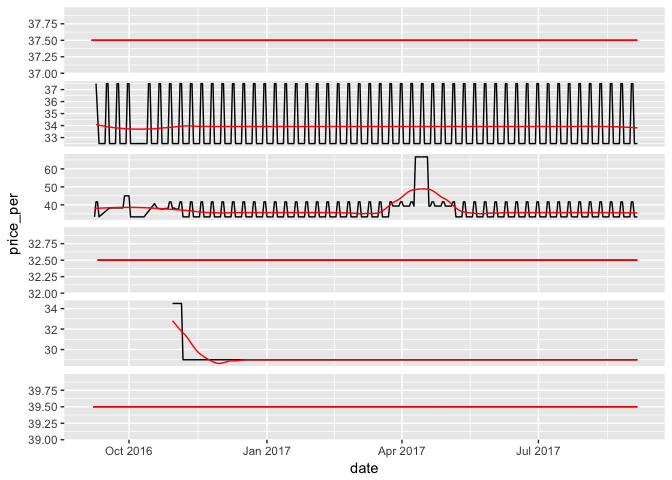Example series with trend models.

``````prices_modeled %>%
ggplot(aes(x = date, group = listing_id)) +
geom_line(aes(y = price_per)) +
geom_line(aes(y = .fitted), color = 'red') +
facet_wrap(~listing_id, ncol = 1, scales = 'free_y') +
theme(strip.text.x = element_blank())``````

As we can see, the `LOESS` smoother is far from a perfect model for the data – there’s more signal left to capture. For now, let’s name the part of the signal that we have captured to reflect the fact that it captures the long-term trend:

``````prices_modeled <- prices_modeled %>%
rename(trend = .fitted)``````

## Summing Up: Nested Models

To wrap things up so far, what did we do to capture the long-term trend? We:

1. Nested our data into a data frame of data frames.
2. We wrote a simple modeling function, whose main feature was that it took a data frame as an argument.
3. We mapped that function onto each of of our little data frames, thereby fitting many models at once.
4. We augmented our data frame to extract the model predictions and residuals in tidy format.
5. We unnested our data so that we could continue to explore and visualize our data.

It’s important to emphasize that the key steps here – model, map, augment – are highly general, and work with many machine learning models, not just `LOESS`. Linear and non-linear regression models, k-means, and singular-value decompositions are just a few of the many models for which the same approach will work smoothly.

In summary,

When you need to fit a model to each group of a data frame, consider using `tidyr::nest`, `purrr::map`, and `broom::augment`.

Why? Because it’s easy to write. Our complete pipeline for fitting 1698 LOESS models to our data is:

``````my_loess <- function(data, span){
loess(price_per ~ date,
data = data,
na.action = na.exclude,
span = span)
}

prices %>%
nest(-listing_id) %>%
mutate(model = map(data, my_loess, span = .5),
preds = map(model, augment)) %>%
unnest(preds)``````

Not bad for a trivial function definition and four lines of code! Clever use of nesting can save you a lot of writing time and a lot of headaches.

# Computing Periodicity

There are plenty of systematic tools in `R` for computing the periodicity of a time series.9 See, for example, `forecast::findfrequency`. However, we have the benefit of knowing the frequency already – by inspection, it’s the seven-day week. We can easily construct the periodic trend using `dplyr` with a little help from `lubridate`, which makes it easy to work with dates in `R`. First, we’ll figure out which day of the week it is, with a little help from `lubridate`.10 `lubridate` is a package for working with dates and times. It fits neatly into the tidy data format.

``````prices_modeled <- prices_modeled %>%
mutate(weekday = wday(date, label = T))

prices_modeled\$weekday[1:5]``````
``````##  Tues Mon  Sun  Sat  Fri
## Levels: Sun < Mon < Tues < Wed < Thurs < Fri < Sat``````

Now that we have a weekday column, we should compute the average per weekday for each listing. But we don’t want to summarise our data down into a smaller data frame – we want the periodic component to simply be another column, that we can compare with our original data directly. The key is to use `group_by() %>% mutate()`. You’ve already seen `group_by() %>% summarise()` – the only difference is that, instead of reducing our data, when we construct the new column each computation will take place “within group”.11 Note that we are averaging the residual, not the original data – since we’ve already captured part of the signal in the `trend` column.

``````prices_modeled <- prices_modeled %>%
group_by(listing_id, weekday) %>%
mutate(periodic = mean(.resid, na.rm = T)) %>%
ungroup() %>%
arrange(listing_id, date)``````

Let’s take a look at our new column:

``prices_modeled %>% select(date, weekday, .resid, periodic)``
``````## # A tibble: 539,046 × 4
##          date weekday       .resid     periodic
##        <date>   <ord>        <dbl>        <dbl>
## 1  2016-09-27    Tues 7.105427e-15 5.945358e-15
## 2  2016-09-28     Wed 7.105427e-15 6.349531e-15
## 3  2016-10-10     Mon 0.000000e+00 5.773160e-15
## 4  2016-10-11    Tues 1.421085e-14 5.945358e-15
## 5  2016-10-17     Mon 7.105427e-15 5.773160e-15
## 6  2016-10-18    Tues 7.105427e-15 5.945358e-15
## 7  2016-10-19     Wed 0.000000e+00 6.349531e-15
## 8  2016-10-20   Thurs 7.105427e-15 5.715235e-15
## 9  2016-10-21     Fri 7.105427e-15 6.642030e-15
## 10 2016-10-22     Sat 7.105427e-15 6.333098e-15
## # ... with 539,036 more rows``````

Just as we’d expect: though the `.resid` column differs between weekdays, the `periodic` column does not.

Now we’ve extracted two kinds of signal from the original data, the `trend` and the `periodic` signals. We can complete our decomposition by constructing a final column, called `remainder`, that’s not captured by the trend signal or the periodic signal:

``````prices_modeled <- prices_modeled %>%
mutate(remainder = .resid - periodic)``````

Let’s visualize this decomposition. We want to visualize four series together:

• The original data, `price_per`
• The seasonal trend, `trend`
• The periodic oscillation, `periodic`
• The remaining signal, `remainder`

This is a good exercise in using `tidyr::gather`: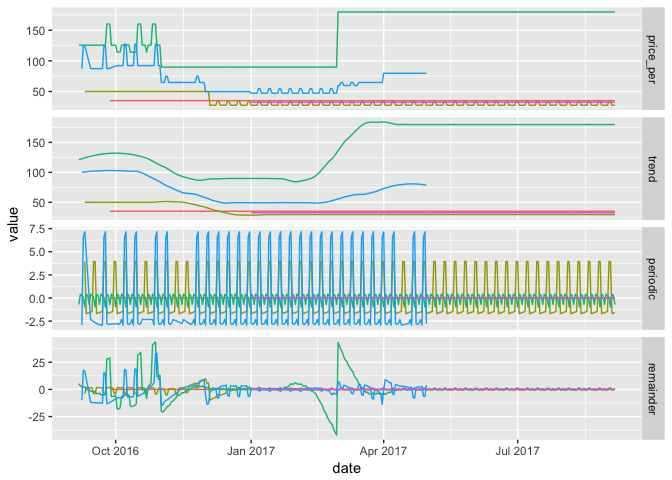Sample decompositions

``````prices_modeled %>%
select(-.se.fit, -.resid, -weekday) %>%
gather(metric, value, -listing_id, -date) %>%
mutate(metric = factor(metric, c('price_per',
'trend',
'periodic',
'remainder'))) %>%
ggplot() +
aes(x = date,
y = value,
group = listing_id,
color = listing_id) +
geom_line() +
facet_grid(metric~., scales = 'free_y') +
guides(color = FALSE)``````

To see what signal is left in the data, we can inspect the average remainder: